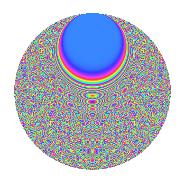# Properties

 Label 1575.2.crLevel 1575 Weight 2 Character orbit cr Rep. character $$\chi_{1575}(16,\cdot)$$ Character field $$\Q(\zeta_{15})$$ Dimension 1888 Sturm bound 480

# Related objects

## Defining parameters

 Level: $$N$$ = $$1575 = 3^{2} \cdot 5^{2} \cdot 7$$ Weight: $$k$$ = $$2$$ Character orbit: $$[\chi]$$ = 1575.cr (of order $$15$$ and degree $$8$$) Character conductor: $$\operatorname{cond}(\chi)$$ = $$1575$$ Character field: $$\Q(\zeta_{15})$$ Sturm bound: $$480$$

## Dimensions

The following table gives the dimensions of various subspaces of $$M_{2}(1575, [\chi])$$.

Total New Old
Modular forms 1952 1952 0
Cusp forms 1888 1888 0
Eisenstein series 64 64 0

## Trace form

 $$1888q + 3q^{2} - 3q^{3} + 235q^{4} - 8q^{5} - 8q^{6} - 8q^{7} - 16q^{8} - 3q^{9} + O(q^{10})$$ $$1888q + 3q^{2} - 3q^{3} + 235q^{4} - 8q^{5} - 8q^{6} - 8q^{7} - 16q^{8} - 3q^{9} - 4q^{10} - 6q^{11} - 23q^{12} - 6q^{13} + 9q^{14} - 42q^{15} + 223q^{16} - 12q^{17} - 6q^{19} - 2q^{20} - 30q^{21} + 2q^{22} - 22q^{23} - 26q^{24} - 8q^{25} + 76q^{26} + 18q^{27} - 20q^{28} - 6q^{29} + 25q^{30} + 3q^{31} + 4q^{32} + 6q^{33} - 10q^{34} - 49q^{35} + 20q^{36} - 6q^{37} - 22q^{38} + 7q^{39} - 22q^{40} - 38q^{41} - 26q^{42} - 16q^{43} + 6q^{44} + 73q^{45} + 2q^{46} + 3q^{47} + 44q^{48} - 8q^{49} - 28q^{50} - 8q^{51} - 14q^{52} + 14q^{53} - 11q^{54} - 34q^{55} - 48q^{56} - 144q^{57} - 46q^{58} - 45q^{59} + 53q^{60} + 3q^{61} - 52q^{62} - 11q^{63} - 436q^{64} + 19q^{65} + 5q^{66} + 3q^{67} - 256q^{68} - 27q^{69} + 8q^{70} - 42q^{71} - 15q^{72} - 6q^{73} - 32q^{74} + 97q^{75} - 16q^{76} - 23q^{77} + 40q^{78} + 15q^{79} + 94q^{80} + q^{81} - 20q^{82} - 48q^{83} - 125q^{84} - 13q^{85} - 22q^{86} - 41q^{87} - 22q^{88} - 39q^{89} - 178q^{90} + 30q^{91} - 30q^{92} + 50q^{93} - 13q^{94} + 18q^{95} + 23q^{96} - 6q^{97} - 142q^{98} - 30q^{99} + O(q^{100})$$

## Decomposition of $$S_{2}^{\mathrm{new}}(1575, [\chi])$$ into newform subspaces

The newforms in this space have not yet been added to the LMFDB.

## Hecke Characteristic Polynomials

There are no characteristic polynomials of Hecke operators in the database